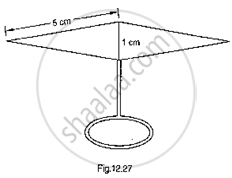# Find the Area of the Blades of Thc Magnetic Compass Shown in Fig.. 12.27. (Take √11 = 3.32). - Mathematics

Find the area of the blades of thc magnetic compass shown in Fig.. 12.27. (Take √11 = 3.32).#### Solution

Area of the blades of magnetic compass = Area of ΔADB + Area of ΔCDB

Semi perimeter (S)= 1/2(5+1+5)=11/2 cm

By using heron’s formulae
Now, area of ΔADB =sqrt(s(s-ad)(s-bd)(s-ba))

sqrt(11/2(11/2-5)(11/2-1)(11/2-5))

=2.49 cm^2

= Also, area of triangle ADB = 𝐴𝑟𝑒𝑎 𝑜𝑓 Δ𝑙𝑒 𝐶𝐷𝐵
∴ Area of the blades of magnetic compass
= 2×(𝑎𝑟𝑒𝑎 𝑜𝑓 Δ𝐴𝐷𝐵)
= 2 × 2.49
= 4.98 m^2

Concept: Area of a Triangle
Is there an error in this question or solution?

#### APPEARS IN

RD Sharma Mathematics for Class 9
Chapter 17 Heron’s Formula
Exercise 17.2 | Q 10 | Page 20
Share